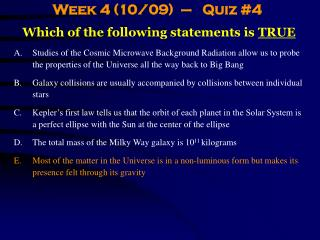DownloadDownload PresentationWhich of the following statements is TRUE

# Which of the following statements is TRUE

Download Presentation## Which of the following statements is TRUE

- - - - - - - - - - - - - - - - - - - - - - - - - - - E N D - - - - - - - - - - - - - - - - - - - - - - - - - - -
##### Presentation Transcript

1. Week 4 (10/09) – Quiz #4 Which of the following statements is TRUE • Studies of the Cosmic Microwave Background Radiation allow us to probe the properties of the Universe all the way back to Big Bang • Galaxy collisions are usually accompanied by collisions between individual stars • Kepler’s first law tells us that the orbit of each planet in the Solar System is a perfect ellipse with the Sun at the center of the ellipse • The total mass of the Milky Way galaxy is 1011 kilograms • Most of the matter in the Universe is in a non-luminous form but makes its presence felt through its gravity

2. Week 4 (10/09) – Quiz #5 Which of the following statements is FALSE • The strong and weak nuclear forces are short-range forces that do not obey the inverse square law • The force of friction is an example of the electromagnetic force • Gravity is the primary force at play during the formation of galaxies • There are five fundamental forces in Nature • The nuclear fusion reactions that power the Sun involve the strong nuclear force

3. Week 4 (10/11) – Quiz #6 Which of the following “Scientist: Fact” associations is the LEAST DIRECT? • Maxwell: Wave nature of electromagnetic radiation • Kepler: The Sun orbits the Milky Way’s center • Newton: Laws of gravity • Einstein: Particle nature of electromagnetic radiation • Kelvin: The motion of molecules ceases at absolute zero

4. Week 4 (10/11) – Quiz #7 Which of the following statements is FALSE? • Einstein showed that the particle nature of electromagnetic radiation can explain the photoelectric effect and was awarded the Nobel Prize for this • Each gamma-ray photon is more energetic than a radio photon and each source of gamma rays is more luminous than a radio source • One of the portions of the electromagnetic spectrum where the atmosphere is transparent (“windows”) closely matches the portion to which the human eye is sensitive and this probably resulted from Darwinian evolution • Scientists have directly measured the tiny fluctuations in the Cosmic Microwave Background Radiation that represent the “seeds” from which galaxies and clusters of galaxies formed • When you look at the student sitting next to you, you are effectively seeing them as they were a fraction of a second in the past

5. Week 4 (10/13) – Quiz #8 Star X has a surface temperature that is 3 times higher than that of the Sun. Both stars have exactly the same radius. Which of the following statements is TRUE? • The luminosity of the Sun is 81 times that of the star X • The typical photon emitted by star X has a lower energy than the typical photon emitted by the Sun • Star X is reddish in color whereas the Sun is yellowish white • If both stars were at the same distance from us, the Sun would appear 81 times fainter than star X • Most of the energy of star X is emitted at a longer wavelength than most of the Sun’s energy

6. Week 4 (10/13) – Quiz #9 Which of the following statements is FALSE: • Kepler’s first law states that the orbit of each planet in our Solar System is an ellipse with the Sun located at one of the foci of the ellipse • We live in an expanding Universe, and yet it is difficult to find a parking space on this campus! • Electrons within atoms can have one of many possible discrete energy levels • Astronomy and astrophysics deal primarily with telling a person’s future from the positions of the planets • Einstein proposed that mass can be converted into energy and vice versa

7. Week 4 (10/13) – Quiz #10 Which of the following statements is TRUE: • The Moon is made of green cheese and the first astronauts who landed on the Moon discovered this very tasty fact • The wave-particle duality of electromagnetic radiation refers to the fact that it can be described as a wave and/or as packets or bundles of energy known as “photons” • Wavelength is directly proportional to frequency in the case of electromagnetic radiation • The luminosity of a black body is proportional to the square of its surface area • The inverse square law states that the flux from a star is inversely proportional to the fourth power of is distance from us